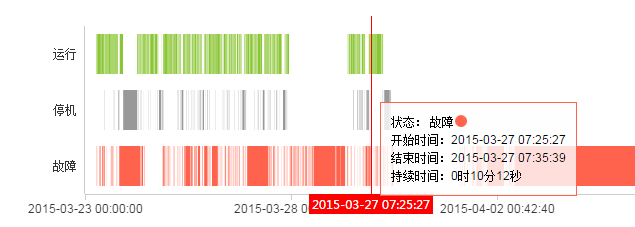echarts甘特图的实现问题1个回答qq_28588927 回复磊落奇伟: 你好，请问您用echarts解决这个问题了么？我也是同样实现您所说的功能呢~麻烦回复一下
3 年多之前 回复3 年多之前 回复Dev的SchedulerControl控件，做甘特图的问题
Android甘特图相关问题，求助

vue+element+dhtmlxGant(甘特图)

dhtmlxgantt甘特图中如何显示序号？

DEV甘特图控件/日程管理控件问题
react怎么开发甘特图?

jquery gantt editor甘特图，json数据中的start和end参数，页面中显示的日期和传输的完全不一致
jquery gantt editor，json数据中的start和end参数，均调整为13位的时间戳。页面中显示的日期和传输的完全不一致， 原因是duration，甘特图会根据开始时间(start)和持续时间(duration)来计算结束日期，计算的结果不包含周六日， 如何让结束日期显示为后台传输的值，而不是显示开始时间和持续时间的计算结果？？？ 急！！！

project 2013与project server 的关系？

DEV 的SchedulerControl控件问题

function [Zp,Y1p,Y2p,Y3p,Xp,LC1,LC2]=JSPGA(M,N,Pm,T,P) %-------------------------------------------------------------------------- %  JSPGA.m %  流水线型车间作业调度遗传算法 %  GreenSim团队原创作品，转载请注明 %  Email:greensim@163.com %  GreenSim团队主页：http://blog.sina.com.cn/greensim %  欢迎访问GreenSim——算法仿真团队→http://blog.sina.com.cn/greensim %-------------------------------------------------------------------------- %  输入参数列表 %  M       遗传进化迭代次数 %  N       种群规模(取偶数) %  Pm      变异概率 %  T       m×n的矩阵，存储m个工件n个工序的加工时间 %  P       1×n的向量，n个工序中，每一个工序所具有的机床数目 %  输出参数列表 %  Zp      最优的Makespan值 %  Y1p     最优方案中，各工件各工序的开始时刻，可根据它绘出甘特图 %  Y2p     最优方案中，各工件各工序的结束时刻，可根据它绘出甘特图 %  Y3p     最优方案中，各工件各工序使用的机器编号 %  Xp      最优决策变量的值，决策变量是一个实数编码的m×n矩阵 %  LC1     收敛曲线1，各代最优个体适应值的记录 %  LC2     收敛曲线2，各代群体平均适应值的记录 %  最后，程序还将绘出三副图片：两条收敛曲线图和甘特图（各工件的调度时序图） %第一步：变量初始化 [m,n]=size(T);%m是总工件数，n是总工序数 Xp=zeros(m,n);%最优决策变量 LC1=zeros(1,M);%收敛曲线1 LC2=zeros(1,N);%收敛曲线2 %第二步：随机产生初始种群 farm=cell(1,N);%采用细胞结构存储种群 for k=1:N X=zeros(m,n); for j=1:n for i=1:m X(i,j)=1+(P(j)-eps)*rand; end end farm{k}=X; end counter=0;%设置迭代计数器 while counter<M%停止条件为达到最大迭代次数 %第三步：交叉 newfarm=cell(1,N);%交叉产生的新种群存在其中 Ser=randperm(N); for i=1:2:(N-1) A=farm{Ser(i)};%父代个体 Manner=unidrnd(2);%随机选择交叉方式 if Manner==1 cp=unidrnd(m-1);%随机选择交叉点 %双亲双子单点交叉 a=[A(1:cp,:);B((cp+1):m,:)];%子代个体 b=[B(1:cp,:);A((cp+1):m,:)]; else cp=unidrnd(n-1);%随机选择交叉点 b=[B(:,1:cp),A(:,(cp+1):n)]; end newfarm{i}=a;%交叉后的子代存入newfarm newfarm{i+1}=b; end %新旧种群合并 FARM=[farm,newfarm]; %第四步：选择复制 FITNESS=zeros(1,2*N); fitness=zeros(1,N); plotif=0; for i=1:(2*N) X=FARM{i}; Z=COST(X,T,P,plotif);%调用计算费用的子函数 FITNESS(i)=Z; end %选择复制采取两两随机配对竞争的方式，具有保留最优个体的能力 Ser=randperm(2*N); for i=1:N f2=FITNESS(Ser(2*i)); if f1<=f2 farm{i}=FARM{Ser(2*i-1)}; fitness(i)=FITNESS(Ser(2*i-1)); else farm{i}=FARM{Ser(2*i)}; end end %记录最佳个体和收敛曲线 minfitness=min(fitness); meanfitness=mean(fitness); LC1(counter+1)=minfitness;%收敛曲线1，各代最优个体适应值的记录 LC2(counter+1)=meanfitness;%收敛曲线2，各代群体平均适应值的记录 pos=find(fitness==minfitness); Xp=farm{pos(1)}; %第五步：变异 for i=1:N if Pm>rand;%变异概率为Pm X=farm{i}; I=unidrnd(m); J=unidrnd(n); X(I,J)=1+(P(J)-eps)*rand; farm{i}=X; end end farm{pos(1)}=Xp; counter=counter+1; end %输出结果并绘图 figure(1); plotif=1; X=Xp; [Zp,Y1p,Y2p,Y3p]=COST(X,T,P,plotif); figure(2); plot(LC1); figure(3); plot(LC2); function [Zp,Y1p,Y2p,Y3p]=COST(X,T,P,plotif) %  JSPGA的内联子函数，用于求调度方案的Makespan值 %  输入参数列表 %  X       调度方案的编码矩阵，是一个实数编码的m×n矩阵 %  T       m×n的矩阵，存储m个工件n个工序的加工时间 %  P       1×n的向量，n个工序中，每一个工序所具有的机床数目 %  plotif  是否绘甘特图的控制参数 %  输出参数列表 %  Zp      最优的Makespan值 %  Y1p     最优方案中，各工件各工序的开始时刻 %  Y2p     最优方案中，各工件各工序的结束时刻 %  Y3p     最优方案中，各工件各工序使用的机器编号 %第一步：变量初始化 [m,n]=size(X); Y1p=zeros(m,n); Y2p=zeros(m,n); Y3p=zeros(m,n); %第二步：计算第一道工序的安排 Q1=zeros(m,1); Q2=zeros(m,1); R=X(:,1);%取出第一道工序 Q3=floor(R);%向下取整即得到各工件在第一道工序使用的机器的编号 %下面计算各工件第一道工序的开始时刻和结束时刻 for i=1:P(1)%取出机器编号 pos=find(Q3==i);%取出使用编号为i的机器为其加工的工件的编号 lenpos=length(pos); if lenpos>=1 Q1(pos(1))=0; if lenpos>=2 for j=2:lenpos Q1(pos(j))=Q2(pos(j-1)); Q2(pos(j))=Q2(pos(j-1))+T(pos(j),1); end end end end Y1p(:,1)=Q1; Y3p(:,1)=Q3; %第三步：计算剩余工序的安排 for k=2:n R=X(:,k);%取出第k道工序 Q3=floor(R);%向下取整即得到各工件在第k道工序使用的机器的编号 %下面计算各工件第k道工序的开始时刻和结束时刻 for i=1:P(k)%取出机器编号 pos=find(Q3==i);%取出使用编号为i的机器为其加工的工件的编号 lenpos=length(pos); if lenpos>=1 EndTime=Y2p(pos,k-1);%取出这些机器在上一个工序中的结束时刻 POS=zeros(1,lenpos);%上一个工序完成时间由早到晚的排序 for jj=1:lenpos POS(jj)=ppp(1); EndTime(ppp(1))=Inf; end %根据上一个工序完成时刻的早晚，计算各工件第k道工序的开始时刻和结束时刻 Q1(pos(POS(1)))=Y2p(pos(POS(1)),k-1); Q2(pos(POS(1)))=Q1(pos(POS(1)))+T(pos(POS(1)),k);%前一个工件的结束时刻 if lenpos>=2 for j=2:lenpos Q1(pos(POS(j)))=Y2p(pos(POS(j)),k-1);%预定的开始时刻为上一个工序的结束时刻 if Q1(pos(POS(j)))<Q2(pos(POS(j-1)))%如果比前面的工件的结束时刻还早 Q1(pos(POS(j)))=Q2(pos(POS(j-1))); end end end end end Y1p(:,k)=Q1; Y2p(:,k)=Q2; Y3p(:,k)=Q3; end %第四步：计算最优的Makespan值 Y2m=Y2p(:,n); Zp=max(Y2m); %第五步：绘甘特图 if plotif for i=1:m for j=1:n mPoint1=Y1p(i,j); mPoint2=Y2p(i,j); mText=m+1-i; PlotRec(mPoint1,mPoint2,mText); Word=num2str(Y3p(i,j)); %text(0.5*mPoint1+0.5*mPoint2,mText-0.5,Word); hold on x1=mPoint1;y1=mText-1; x2=mPoint2;y2=mText-1; x4=mPoint1;y4=mText; %fill([x1,x2,x3,x4],[y1,y2,y3,y4],'r'); fill([x1,x2,x3,x4],[y1,y2,y3,y4],[1,0.5,1]); text(0.5*mPoint1+0.5*mPoint2,mText-0.5,Word); end end end function PlotRec(mPoint1,mPoint2,mText) %  此函数画出小矩形 %  输入: %  mPoint1    输入点1,较小,横坐标 %  mPoint2    输入点2,较大,横坐标 %  mText      输入的文本,序号,纵坐标 vPoint = zeros(4,2) ; vPoint(1,:) = [mPoint1,mText-1]; vPoint(2,:) = [mPoint2,mText-1]; vPoint(3,:) = [mPoint1,mText]; vPoint(4,:) = [mPoint2,mText]; plot([vPoint(1,1),vPoint(2,1)],[vPoint(1,2),vPoint(2,2)]); hold on ; plot([vPoint(1,1),vPoint(3,1)],[vPoint(1,2),vPoint(3,2)]); plot([vPoint(2,1),vPoint(4,1)],[vPoint(2,2),vPoint(4,2)]); plot([vPoint(3,1),vPoint(4,1)],[vPoint(3,2),vPoint(4,2)]);
DEV的schedulerControl问题

Flex多函数同时被调用时，执行顺序？

EXTJS4 tree为什么不显示子菜单
![图片说明](https://img-ask.csdn.net/upload/201603/04/1457080911_470892.png) "treeJson":[{"id":1,"text":"个人中心","children":[ {"id":3,"text":"新建任务","leaf":true,"checked":false}, {"id":4,"text":"我的签核","leaf":true,"checked":false}, {"id":5,"text":"我的申请","leaf":true,"checked":false}, {"id":6,"text":"我的待办","leaf":true,"checked":false}, {"id":7,"text":"甘特图","leaf":true,"checked":false}, {"id":8,"text":"我的已办","leaf":true,"checked":false}, {"id":9,"text":"日常工作","leaf":true,"checked":false}, {"id":10,"text":"我的收藏","leaf":true,"checked":false}, {"id":11,"text":"通讯录","leaf":true,"checked":false}],"leaf":true,"checked":false}, {"id":22,"text":"知识库","children":[],"leaf":true,"checked":false}, {"id":23,"text":"其他系统","children":[],"leaf":true,"checked":false}, {"id":24,"text":"系统管理","children":[ {"id":26,"text":"人员管理","leaf":true,"checked":false}, {"id":27,"text":"系统消息","leaf":true,"checked":false}, {"id":28,"text":"菜单管理","leaf":true,"checked":false}, {"id":29,"text":"在线用户","leaf":true,"checked":false}, {"id":30,"text":"权限管理","leaf":true,"checked":false}, {"id":32,"text":"流程管控","leaf":true,"checked":false}],"leaf":true,"checked":false}, {"id":25,"text":"帮助中心","children":[],"leaf":true,"checked":false}] ``` { id:'sysTree', xtype: 'treepicker', fieldLabel: '菜单', name : 'tid', displayField: 'text', valueField: 'id', minPickerHeight: 200, //最小高度，不设置的话有时候下拉会出问题 editable: true, //启用编辑，主要是为了清空当前的选择项 enableKeyEvents: true, //激活键盘事件 cascade : 'child',//级联方式:1.child子级联(仅向下勾选子节点)；2.parent父级联(仅向上勾选父节点)；3.both全部级联(向上向下的父/子节点均会勾选) checkModel:'single',//当json数据为不带checked的数据时只配置为single,带checked配置为double为单选,不配置为多选 store: Ext.create('Ext.data.TreeStore',{ fields: ['id','text'], root: { id:0, text: '全部', expanded: true, checked: false }, proxy: { type: 'ajax', url: 'treeAction_checkTree.action', reader: { type: 'json', root : 'treeJson' } }, autoLoad: true }), listeners: { keyup : function(){ //鼠标弹起时清空所有的值 Ext.getCmp("sysTree").setValue(""); } } } ```
130 个相见恨晚的超实用网站，一次性分享出来

win10系统安装教程（U盘PE+UEFI安装）

《奇巧淫技》系列-python！！每天早上八点自动发送天气预报邮件到QQ邮箱

Linux(服务器编程):15---两种高效的事件处理模式（reactor模式、proactor模式）

HAL 硬件抽象层介绍

11月8日，由中国信息通信研究院、中国通信标准化协会、中国互联网协会、可信区块链推进计划联合主办，科技行者协办的2019可信区块链峰会将在北京悠唐皇冠假日酒店开幕。 　　区块链技术被认为是继蒸汽机、电力、互联网之后，下一代颠覆性的核心技术。如果说蒸汽机释放了人类的生产力，电力解决了人类基本的生活需求，互联网彻底改变了信息传递的方式，区块链作为构造信任的技术有重要的价值。 　　1
8年经验面试官详解 Java 面试秘诀
作者 | 胡书敏 责编 | 刘静 出品 | CSDN（ID：CSDNnews） 本人目前在一家知名外企担任架构师，而且最近八年来，在多家外企和互联网公司担任Java技术面试官，前后累计面试了有两三百位候选人。在本文里，就将结合本人的面试经验，针对Java初学者、Java初级开发和Java开发，给出若干准备简历和准备面试的建议。   Java程序员准备和投递简历的实

Linux(内核剖析):19---中断总体概述

Java工作4年来应聘要16K最后没要,细节如下。。。

CPU对每个程序员来说，是个既熟悉又陌生的东西？ 如果你只知道CPU是中央处理器的话，那可能对你并没有什么用，那么作为程序员的我们，必须要搞懂的就是CPU这家伙是如何运行的，尤其要搞懂它里面的寄存器是怎么一回事，因为这将让你从底层明白程序的运行机制。 随我一起，来好好认识下CPU这货吧 把CPU掰开来看 对于CPU来说，我们首先就要搞明白它是怎么回事，也就是它的内部构造，当然，CPU那么牛的一个东...

Python实战：抓肺炎疫情实时数据，画2019-nCoV疫情地图

NO.1 　有20瓶药丸，其中19瓶装有1克/粒的药丸，余下一瓶装有1.1克/粒的药丸。给你一台称重精准的天平，怎么找出比较重的那瓶药丸？天平只能用一次。 解法 有时候，严格的限制条件有可能反倒是解题的线索。在这个问题中，限制条件是天平只能用一次。 因为天平只能用一次，我们也得以知道一个有趣的事实：一次必须同时称很多药丸，其实更准确地说，是必须从19瓶拿出药丸进行称重。否则，如果跳过两瓶或更多瓶药...